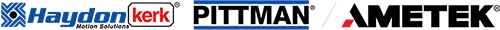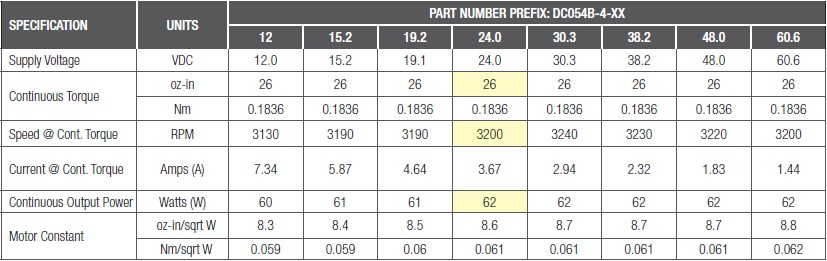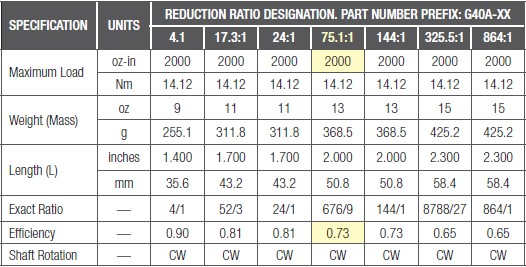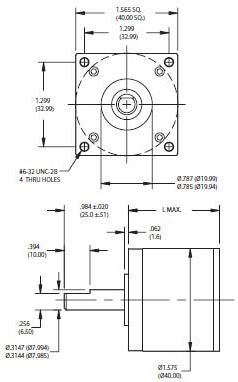# Finding the Right Motor Gearbox Combination to Optimize Performance

What are the steps necessary to select the right motor-gearbox combination for an application and calculate its final torque and speed to help predict performance? When paired with a motor, gearboxes offer a number of mechanical and economic advantages. For instance, they increase output torque while reducing output speed to improve operational efficiency and overall performance. They help match motor inertia to load inertia to optimize system response. And they offer a cost-effective and compact solution for space-constrained applications.

Typically, it is advantageous to incorporate a gearbox with a motor when system speeds are low and torques are high. Low speeds are defined as anything under 100 RPM (about 10.5 rad/sec), and high torques are defined as those over 100 oz-in (about 0.706 Newton-meters).Selecting a Motor
With numerous motors to choose from, each offering different capabilities and characteristics, it’s important to determine which one will best suit your application’s requirements. A few motors to consider include permanent magnet DC (PMDC) brush and brushless motors, stepper motors, AC induction motors and synchronous AC motors.

 Brush motors are typically cost-effective but are limited by how long their brushes can last in applications. Brushless motors aren’t dependent on brush lifecycles, but tend to cost more. DC motors perform well in servo applications, while AC motors are a good option in non-servo applications.Selecting a Gearbox
Like motors, gearbox selection also requires careful consideration. With various gearbox choices, including spur, planetary and right angle, think about the following questions when making a decision:

 How much backlash does the gearbox have? Can the gearbox be back driven by the load? Can the gearbox handle heavy axial and/or radial loads required by the application? Does the selected gearbox meet the application’s size constraints?Calculating Torque and Speed: An Example
After considering the above and selecting the appropriate motor and gearbox for an application, it’s time to start calculating. The first step is determining how much output power is needed. To do this, multiply torque by speed. In this example the torque is expressed in oz-in and speed in RPM. For these units, use a multiplying factor of 7.4 x 10-4 to yield the appropriate watts output. To satisfy the low speed/high torque criteria stated earlier (under 100 RPM and torque over 100 oz-in [0.706 Nm]), let’s use 40 RPM at a torque of 1400 oz-in. With these numbers, the output power required by the device is calculated as follows:

Output Power = 40 x 1400 x 7.4 x 10-4 = 41.44 watts

Next, determine the rated motor power by multiplying the above power output by 150%, which is a good starting point when trying to allow for gearbox friction and operational efficiency in a calculation.

Rated Motor Power = 41.44 x 1.5 = 62.16 watts

Now it’s time to consider and select specific components for this example application. Based on the criteria described in “Selecting a Motor,” the PMDC brush motor was selected for the application in this example. This example also uses AMETEK Pittman solutions because there are both brush and brushless motors to choose from, as well as spur and planetary gearboxes.Figure 1: Brush Commutated DC Servo Motor Data Sheet, Part Number DC054B-4 The smallest unit offered is a DC054B-4 (14204), rated at 62 watts continuous output power, as shown above.Figure 2: To select the gearbox, find all those listed that have a continuous torque rating of at least 1400 oz-in. One such gearbox is the G40A planetary series, shown above.Now that a motor and gearbox have been selected, it’s time to see if these components provide the desired torque and speed. First, find the rated speed of the selected motor in Figure 1, listed at 3200 RPM. To find the required gear ratio, divide the motor speed of 3200 RPM by the required output speed of 40 RPM.

Ratio = 3200 / 40 = 80:1

The nearest ratio to this in Figure 2 is 75.1:1, which is within 95% of the desired value and should work for an initial attempt.

To calculate the torque this motor-gearbox combination will produce, multiply the motor continuous torque, see Figure 1, by the gear ratio and gearbox efficiency, see Figure 2, as follows:

Torque = 26 x 75.1 x 0.73 = 1425 oz-in

The calculated torque meets the stated value of 1400 oz-in and is very close to the full-rated motor torque, which means the associated motor speed will be 3200 RPM. To get the final gearbox output speed, perform the following calculation:

Speed = 3200 / 75.1 = 42.6 RPM

Based on these calculations, the selected motor-gearbox combination will provide the application’s desired torque and speed.In Conclusion
It’s important to remember that these calculated values are approximate and considerations might need to be made to account for motor parameter variations. Still, the same basic concepts and calculations should apply.

Additionally, please note that this example assumes a continuously running application (100% duty cycle). If the duty cycle is less than 100%, then a smaller motor could also meet the application’s need. For instance, if the actual usage produces speed and torque for only 25% of the time, perhaps on for one second and off for three seconds, then the new RMS torque (continuous torque) can be calculated as follows:

RMS Torque = (14002 / 4)½ = 700 oz-in

Reflecting this torque through the 75.1:1 gearbox yields a motor torque of:

Motor Torque = 700 / 75.1 / 0.73 = 12.77 oz-in

This is approximately half the rated motor torque necessary for 100% duty cycle applications (see Figure 1), thus allowing use of a smaller motor unit to reduce cost and save space. The gearbox, however, must remain the same because the torque (1400 oz-in) capability is still necessary when the application is running to prevent gear breakage.

Taking each of these factors into account plays a key role in determining the motor-gearbox combination that will help applications and systems run more efficiently and more cost-effectively.